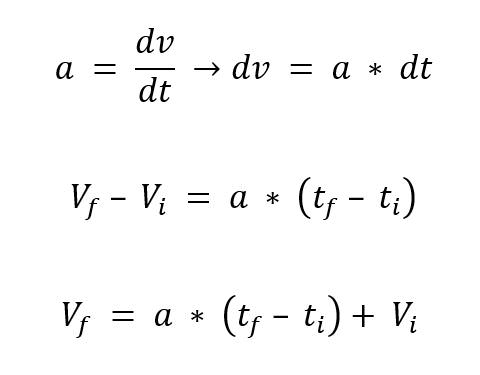# [Help] BNO055 Acceleration/Velocity data!

Hello,

I am a beginner user of Arduino and i needed help with my school project.

I am trying to estimate the velocity using BNO55 sensor.

With the help of manual instructions provided, i was able to create a sketch that determines the linear acceleration using the following lines:

``````imu::Vector<3> euler = bno.getVector(Adafruit_BNO055::VECTOR_LINEARACCEL);

Serial.print(millis());
Serial.print("\t\t");
Serial.print("X: ");
Serial.print(euler.x());
Serial.print("\t\t");
Serial.print(" Y: ");
Serial.print(euler.y());
Serial.print(" Z: ");
Serial.print(euler.z());
Serial.print("\t\t");
``````

• The question is, how to calculate the velocity using the linear acceleration data that the sensor outputs?

any ideas/recommendations?

thanks!

The first question is, how to calculate the velocity using the linear acceleration data that the sensor outputs?

You integrate the acceleration with respect to time.

For a short time interval, (change in velocity) = (acceleration)*(time interval)

The acceleration has to be in units of meters/second^2 and time in seconds to get velocity in meters/second.

is that what you meant?I tried doing so, but the issue is that the values that i get are in-consistent?

in other words, let us assume that i get into my vehicle, and go from 0 to 20 mph within 10 seconds in reality, the results that i get are not near that.

the values that i am getting are spiking up and down.

Assuming your measurement units are correct (although you have not provided any evidence for that), you are discovering why this approach does not work very well at all.

yes, I've converted the units from mph to m/s.

also, the website that you shared is very useful, i don't really mind having inaccurate results as long as i have the right approach to the matter.

yes, i do understand there will be setbacks of using BNO55 to such project, but i am forced to do so..

according to the table in the website, the acceleration data was increasing subsequently, he had no negative readings, does that mean i am doing something wrong mathematically? software? or hardware issue?

again, thanks for the quick replies!!

yes, I've converted the units from mph to m/s.

does that mean i am doing something wrong mathematically? software? or hardware issue?

Additionally, you have failed to read and follow the directions in the "How to use this forum post".

huge apologies with regards the terrible post, i'll fix it.

Can you please elaborate more on units? what do you mean by stating